# Test: Calculus- 1 - Computer Science Engineering (CSE)

Test Description

## 20 Questions MCQ Test GATE Computer Science Engineering(CSE) 2024 Mock Test Series - Test: Calculus- 1

Test: Calculus- 1 for Computer Science Engineering (CSE) 2023 is part of GATE Computer Science Engineering(CSE) 2024 Mock Test Series preparation. The Test: Calculus- 1 questions and answers have been prepared according to the Computer Science Engineering (CSE) exam syllabus.The Test: Calculus- 1 MCQs are made for Computer Science Engineering (CSE) 2023 Exam. Find important definitions, questions, notes, meanings, examples, exercises, MCQs and online tests for Test: Calculus- 1 below.
Solutions of Test: Calculus- 1 questions in English are available as part of our GATE Computer Science Engineering(CSE) 2024 Mock Test Series for Computer Science Engineering (CSE) & Test: Calculus- 1 solutions in Hindi for GATE Computer Science Engineering(CSE) 2024 Mock Test Series course. Download more important topics, notes, lectures and mock test series for Computer Science Engineering (CSE) Exam by signing up for free. Attempt Test: Calculus- 1 | 20 questions in 60 minutes | Mock test for Computer Science Engineering (CSE) preparation | Free important questions MCQ to study GATE Computer Science Engineering(CSE) 2024 Mock Test Series for Computer Science Engineering (CSE) Exam | Download free PDF with solutions
 1 Crore+ students have signed up on EduRev. Have you?
Test: Calculus- 1 - Question 1

### Let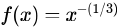and  denote the area of region bounded by f(x) and the X-axis, when  varies from -1 to 1. Which of the following statements is/are TRUE? I.  is continuous in [-1, 1] II.  is not bounded in [-1, 1] III.  is nonzero and finite

Detailed Solution for Test: Calculus- 1 - Question 1

I. False.
II. True.
III. True. An area is always positive, while the definite integral might be composed of several regions, some positive and some negative. A definite integral gets you the net area, because any part of the graph that is below the x-axis will give you a negative area. So, a definite integral is not necessarily the area under the curve, but the value of the area above the x-axis less the area under the x-axis. So, A is non-zero and finite.

Test: Calculus- 1 - Question 2

### The function y=|2 - 3x|

Detailed Solution for Test: Calculus- 1 - Question 2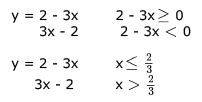as y is polynomial it is continuous and differentiable at all points but don't know at = 2/3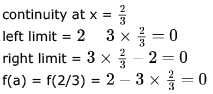LL = RL = f(a) so y is continuous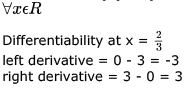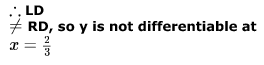Test: Calculus- 1 - Question 3

### Which one of the following functions is continuous at x = 3?

Detailed Solution for Test: Calculus- 1 - Question 3

For continuity, Left hand limit must be equal to right hand limit. For continuity at x = 3, the value of f(x) just above and just below 3 must be the same.

A. f(3) = 2. f(3+) = x - 1 = 2. f(3-) = (x+3)/3 = 6/3 = 2. Hence continuous.
B. f(3) = 4. f(3+) = f(3-) = 8 - 3 = 5. So, not continuous.
C. f(3) = f(3-) = x + 3 = 6. f(3+) = x - 4 = -1. So, not continuous.
D. f(3) is not existing. So, not continuous.

Test: Calculus- 1 - Question 4

A function f(x) is continuous in the interval [0,2]  f(0) = f(2) = -1 and f(1) = 1.  Which one of the following statements must be true?

Detailed Solution for Test: Calculus- 1 - Question 4

Let's define a new function g,
g(y) = f(y) -f(y+1)
Since function f is continuous in [0,2], therefore g would be continuous in [0,1] g(0) = -2, g(1) = 2
since g is continuous and goes from negative to positive value in [0,1]. therefore at some point g would be 0 in (0,1).
g=0 ⇒ f(y) = f(y+1) for some y in (0,1)

Therefore, correct answer would be (A).

Test: Calculus- 1 - Question 5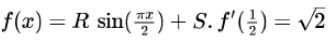and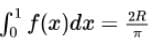, then the constants  and  are

Detailed Solution for Test: Calculus- 1 - Question 5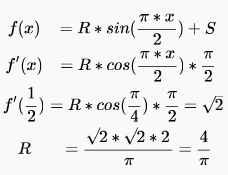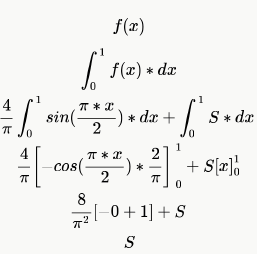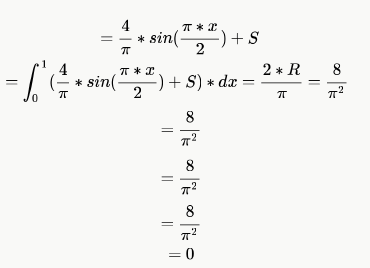*Answer can only contain numeric values
Test: Calculus- 1 - Question 6

Let f(x) be a polynomial and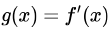be its derivative. If the degree of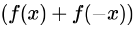is 10, then the degree of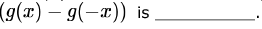Detailed Solution for Test: Calculus- 1 - Question 6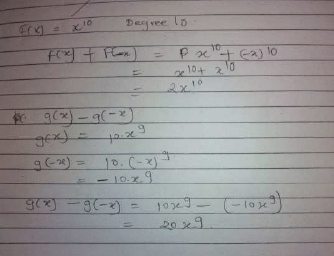F is some function where the largest even degree term is having degree  10. no restiction on odd degree terms.
since f(x)+f(-x)= degree 10
even power gets converted to odd in derivative.
then the the degrre of required expression =9.
the odd powers in F will become even in derivative and G(X)-G(-X) retains only odd powers.

Test: Calculus- 1 - Question 7

Consider the following two statements about the function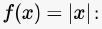• P. f(x) is continuous for all real values of .
• Q .f(x) is differentiable for all real values of  .

Which of the following is TRUE?

Detailed Solution for Test: Calculus- 1 - Question 7

f(x)=|x| here for all values of x, f(x) exists. therefore it is continuous for all real values of x. At x=0, f(x) is not differentiable. Because if we take the left hand limit here, it is negative while the right hand limit is positive.

Test: Calculus- 1 - Question 8

Consider the function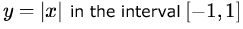. In this interval, the function is

Detailed Solution for Test: Calculus- 1 - Question 8

It is continuous but not differentiable at x=0 as left hand limit will be negative while the right hand limit will be positive but for differentiation, both must be same.

*Answer can only contain numeric values
Test: Calculus- 1 - Question 9

Let f be a function defined by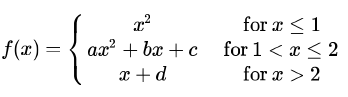Find the values for the constants a, b,c  and d so that f is continuous and differentiable everywhere on the real line.

Detailed Solution for Test: Calculus- 1 - Question 9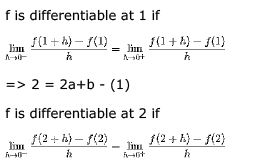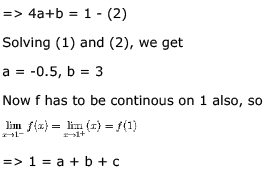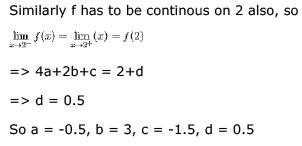Test: Calculus- 1 - Question 10

Let the function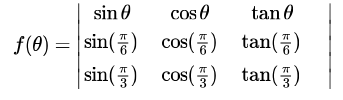where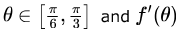denote the derivative of f with respect to θ. Which of the following statements is/are TRUE?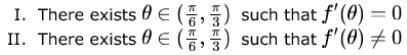Detailed Solution for Test: Calculus- 1 - Question 10

We need to solve this by rolle's theorem, to apply rolle's theorem following 3 conditions should be satisfied:
1) f(x) should be continuous in interval [a, b],
2) f(x) should be differentiable in interval (a, b), and 3) f(a) = f(b)
If these 3 conditions are satisfied simultaneously then, there exists at least one 'x' such that f '(x) = 0
So, for the above question, it satisfies all the three conditions, so we can apply rolle's theorem, i.e, there exists 'at least one' theta that gives f '(theta) = 0
Also, the given function is also not a constant function, i.e f '(theta) ≠ 0

*Answer can only contain numeric values
Test: Calculus- 1 - Question 11

The function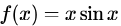satisfies the following equation: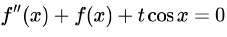. The value of  is______.

Detailed Solution for Test: Calculus- 1 - Question 11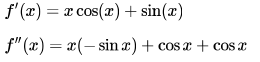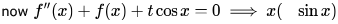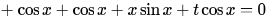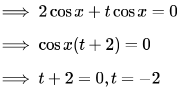Test: Calculus- 1 - Question 12

The formula used to compute an approximation for the second derivative of a function f at a point x0 is

Test: Calculus- 1 - Question 13

In the interval  [0,π] the equation x = cos x has

Detailed Solution for Test: Calculus- 1 - Question 13

f you consider x=0 then cosx=1
now if x= PI/4 = 0.785 then cosx=0.7071
for some x value x=cosx
after this x is increasing and cosx is decreasing. so we can say exactly 1 solution.

EDIT-
It is very easy to show that the equation x = cos x has a unique solution. For example take f(x) = c - cos x and notice that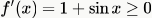(equality holding in isolated points) so f(x) is strictly increasing and hence the equation can have at most one solution.

At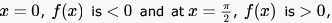and function is continious (difference of two continuous functions is continuous). Therefore there is solution in ,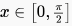hence there is a solution in [0,π]

Test: Calculus- 1 - Question 14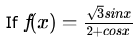then the range of f(x) is

Detailed Solution for Test: Calculus- 1 - Question 14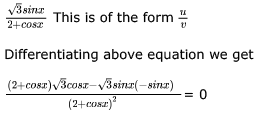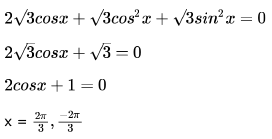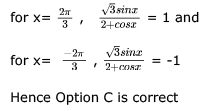Test: Calculus- 1 - Question 15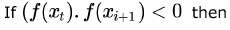Detailed Solution for Test: Calculus- 1 - Question 15

as f(xi).f(xi+1) <0
means one of them is positive and one of them in negative . as their multiplication is negative
so when you draw the graph for f(x) where xi<=x<=xi+1. Definitely F(x) will cut the X- axis.
so there will definitely a root of F(x) between xi and xi+1

Test: Calculus- 1 - Question 16

If  f(x) = x2 and g(x) = x sinx +cosx then

Detailed Solution for Test: Calculus- 1 - Question 16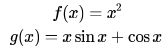We are asked to make this two function equal and see at how many points they meet.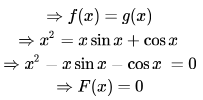This F(x) is even and we just need to check at how many points F(x) crosses the X - axis for x > 0.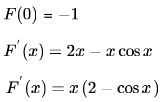Here 2-cos x is greater than 0 and  (2 - cosx) will be also greater than  for x > 0.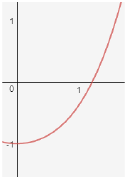As we have already seen that F(x) is even, therefore F(x) has only two real roots.

Test: Calculus- 1 - Question 17

Given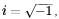what will be the evaluation of the definite integral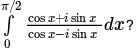Detailed Solution for Test: Calculus- 1 - Question 17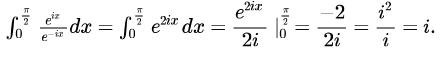Test: Calculus- 1 - Question 18

What is the value of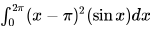Detailed Solution for Test: Calculus- 1 - Question 18

Put  x - π = t then limit 0 changes to  - π and upper limit 2π changes to π.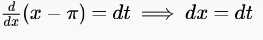Integration of  t2 for limit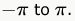to . One is an odd function and one is even and product of odd and even functions is odd function and integrating an odd function from the same negative value to positive value gives 0.

Test: Calculus- 1 - Question 19

If for non-zero x,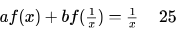where a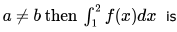Detailed Solution for Test: Calculus- 1 - Question 19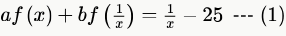Integrating both sides,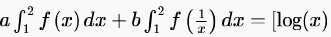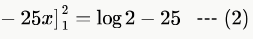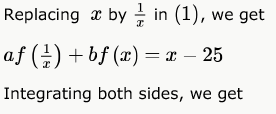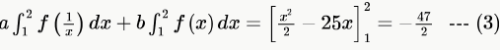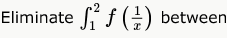(2) and (3) by multiplying (2) by  and (3) by  and subtracting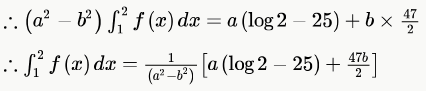Test: Calculus- 1 - Question 20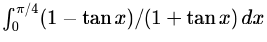Detailed Solution for Test: Calculus- 1 - Question 20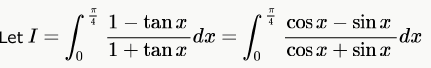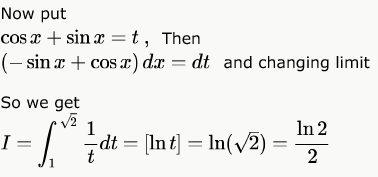## GATE Computer Science Engineering(CSE) 2024 Mock Test Series

151 docs|216 tests
Information about Test: Calculus- 1 Page
In this test you can find the Exam questions for Test: Calculus- 1 solved & explained in the simplest way possible. Besides giving Questions and answers for Test: Calculus- 1, EduRev gives you an ample number of Online tests for practice

## GATE Computer Science Engineering(CSE) 2024 Mock Test Series

151 docs|216 tests